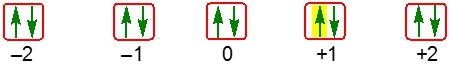Start typing, then use the up and down arrows to select an option from the list.## General Chemistry

Learn the toughest concepts covered in Chemistry with step-by-step video tutorials and practice problems by world-class tutors

9. Quantum Mechanics

# Quantum Numbers: Spin Quantum Number

The Spin Quantum Number deals with the spin of an electron within an orbital.

Spin Quantum Number
1
concept

## Quantum Numbers: Spin Quantum Number1m
Play a video:
with the spin quantum number. We actually look at the individual spins of the electrons within a given orbital. We're gonna say an orbital can hold a maximum of two electrons that have opposite spins according to the poly exclusion principle, not the poly exclusion principle says that no two electrons within the same orbital can have the same four quantum numbers by having opposite spins that affect their spin quantum number, which is M sebas. Now it deals with the rotational spin of an electron inside an atomic orbital. We're going to say here that the way we do it is we start out feeling in orbital with an electron that points up followed by the next one Pointing down. This indicates that each electron has an opposite spin. We're gonna say an electron that points up has an M s value M sub s value off plus one half and one that points down has an M. C s value of negative one half. So here we have our illustration of an orbital in red and we have those two electrons within that orbital one is spinning up the first one and then one is spinning down. So Just remember when it comes to the spin of an electron within an orbital, we look at the subs, quantum number.
2
example

## Quantum Numbers: Spin Quantum Number Example 11m
Play a video:
here we need to provide the n, l, M sable and SMS quantum numbers for the two highlighted electrons in a three D principal level. All right, so because they told us it's a three D principal level that means n equals three. Next, we're going to see that our M. Sobel remember, That's the range is negative. One positive one M. Sobel is the range of l. So that would mean that L is equal to one. We're looking specifically at electrons in the orbital marked zero, so that EMS able for both electrons, is zero. Now, though, one electron is pointing up and one is pointing down that would give us two different M cfs quantum numbers. So let's call this electron one for this electron here. Since it's spinning up, it's M subs would be plus one half and then for electron to the one that's pointing down. It would have the same N l and M slash l, but because it's pointing down, it would be minus one half. So these will be the four quantum numbers for the two electrons within this given orbital
3
Problem

Select the correct quantum numbers for the highlighted electrons in a set of 5d orbitals.4
Problem

Which of the following set of quantum numbers is possible?

5
Problem

Which of the following set of quantum numbers is possible for an electron in a set of 6f orbitals?

6
Problem

Which of the following statements is false?